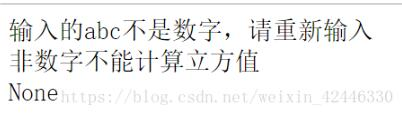# python自定义函数实现一个数的三次方计算方法

python自定义函数在运行时，最初只是存在内存中，只有调用时才会触发运行。defcube_count(a):i

python自定义函数在运行时，最初只是存在内存中，只有调用时才会触发运行。

``````
def cube_count(a):
if is_number(a):
return a**3
else:
print("非数字不能计算立方值")

def is_number(a):
if not isinstance(a,(int,float)):
print("输入的%s不是数字，请重新输入"%a)
return False
else:
return True
c = cube_count("abc")
print(c)````````````
c=cube_count(10)
print(c)``````

python 自定义函数 三次方